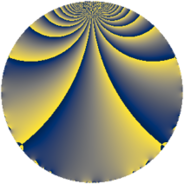# Properties

 Label 385.2.cLevel $385$ Weight $2$ Character orbit 385.c Rep. character $\chi_{385}(76,\cdot)$ Character field $\Q$ Dimension $32$ Newform subspaces $1$ Sturm bound $96$ Trace bound $0$

# Related objects

## Defining parameters

 Level: $$N$$ $$=$$ $$385 = 5 \cdot 7 \cdot 11$$ Weight: $$k$$ $$=$$ $$2$$ Character orbit: $$[\chi]$$ $$=$$ 385.c (of order $$2$$ and degree $$1$$) Character conductor: $$\operatorname{cond}(\chi)$$ $$=$$ $$77$$ Character field: $$\Q$$ Newform subspaces: $$1$$ Sturm bound: $$96$$ Trace bound: $$0$$

## Dimensions

The following table gives the dimensions of various subspaces of $$M_{2}(385, [\chi])$$.

Total New Old
Modular forms 52 32 20
Cusp forms 44 32 12
Eisenstein series 8 0 8

## Trace form

 $$32q - 28q^{4} - 36q^{9} + O(q^{10})$$ $$32q - 28q^{4} - 36q^{9} + 6q^{11} + 8q^{14} - 4q^{15} + 36q^{16} - 40q^{22} + 16q^{23} - 32q^{25} + 68q^{36} + 32q^{37} - 24q^{42} + 36q^{44} - 8q^{53} - 80q^{56} - 40q^{60} - 36q^{64} - 32q^{67} - 28q^{70} + 64q^{77} + 168q^{78} - 64q^{86} + 116q^{88} + 36q^{91} - 104q^{92} - 88q^{93} - 44q^{99} + O(q^{100})$$

## Decomposition of $$S_{2}^{\mathrm{new}}(385, [\chi])$$ into newform subspaces

Label Dim. $$A$$ Field CM Traces $q$-expansion
$$a_2$$ $$a_3$$ $$a_5$$ $$a_7$$
385.2.c.a $$32$$ $$3.074$$ None $$0$$ $$0$$ $$0$$ $$0$$

## Decomposition of $$S_{2}^{\mathrm{old}}(385, [\chi])$$ into lower level spaces

$$S_{2}^{\mathrm{old}}(385, [\chi]) \cong$$ $$S_{2}^{\mathrm{new}}(77, [\chi])$$$$^{\oplus 2}$$